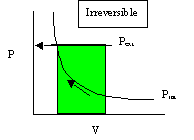# 19.2: Pressure-Volume Work

Work in general is defined as a product of a force F and a path element ds. Both are vectors and work is computed by integrating over their inproduct

$w = \int \textbf{F} \cdot \textbf{ds}$

Moving an object against the force of friction as done in the above dissipation experiment is but one example of work.

$w_{friction} = \int \textbf{F}_{friction} \cdot \textbf{ds}$

We could also think of electrical work. In that case we would be moving a charge e (e.g. the negative charge of an electron) against an electrical (vector) field $$\textbf{E}$$. The work would be:

$w_{electical} = \int e \textbf{E} \cdot \textbf{ds}$

Other examples are the stretching of a rubber band against the elastic force or moving a magnet in a magnetic field etc, etc.

#### A special case: volume work

In the case of a cylinder we can introduce the area of the piston A and forget about the vectorial nature The movement of the piston is constrained to one direction, the one in which we apply pressure (P being force F per area A). Besides: the molecules of the gas rapidly equilibrate to the applied pressure and make it into an all-sided phenomenon.

$w_{volume} = \int \left( \dfrac{F}{\cancel{A}} \right) (\cancel{A}\,ds) = \int P\,dv \label{Volume work}$

This particular form of work is called volume work and will play an important role in the development of our theory. Notice however that volume work is only one form of work.

Sign conventions

It is important to create a sign convention at this point: positive heat, positive work is always what you put in into the system. If the system decides to give of heat or work that gets a minus sign.

In other words: you pay the bill.

To comply with this convention we need to rewrite volume work (Equation $$\ref{Volume work}$$) as

$w_{volume} = - \int \left( \dfrac{F}{\cancel{A}} \right) (\cancel{A}\,ds) = - \int P\,dv$

Hence, to decrease the volume of the gas ($$\Delta V$$ is negative), we must put in (positive) work

## Compressing a gas in a cylinder

Thermodynamics would not have come very far without cylinders to hold gases, in particular steam. Look at fig 19.1 to see a cylinder-based version of the previous statement. Also go through the argument on page 767 to see that we can compute one type of work that is very important for gases, the volume work as follows:

$w_{volume} = -P_{ext}ΔV \label{PV work}$

Equation $$\ref{PV work}$$ only holds under isobaric conditions. If the outside pressure changes during compression we must integrate over all contributions:

$w = - \int_{V_{state}}^{V_{finish}}P_{ext}dV$

Note that the minus sign in Equation $$\ref{PV work}$$ is consistent with the "you pay principle". When the volume gets smaller $$ΔV$$ is negative and $$w$$ is positive. This is the work you have to put in.

Let us compare two ways (paths) of compressing a gas from volume 1 to volume 2.

### Compression one (irreversible)

In a P-V diagram of an ideal gas P is a hyperbolic function of V see fig 19.2, but this refers to the internal pressure of the gas. It is the external one that counts when computing work and they are not necessarily the same. As long as Pextermal is constant, work is represented by a rectangle. But how can the external pressure be different from the internal one?1. Start with cylinder 1 liter, both external and internal pressure 1 bar.
2. Peg the piston in a fixed position
3. Put cylinder in a pressure chamber with Pext= 2 bar.
Now you are ready to do the experiment:
4. Suddenly pull the peg

The piston will shoot down till the internal and external pressures balance out again and the volume is 1/2 L. Notice that the external pressure was maintained constant at 2 bar during the peg-pulling and that the internal and external pressures were not balanced at all time.